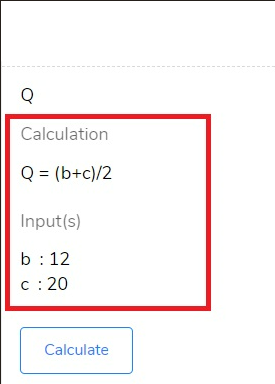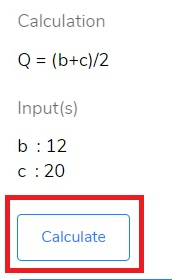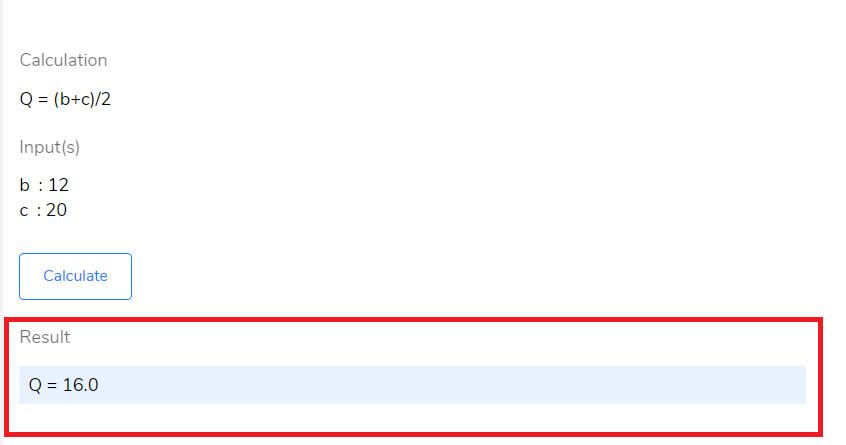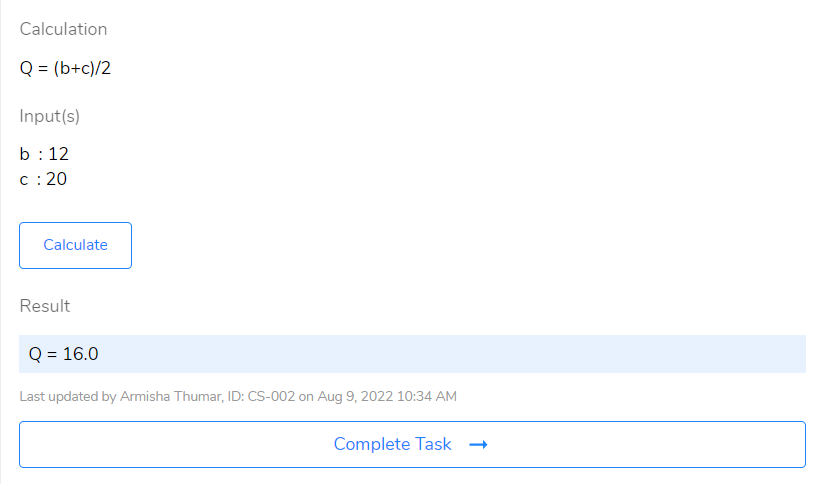# How to execute a Calculation activity?

## Click on Calculate button to auto calculate the results as per the configured formula.

Steps to perform calculation activity:

1. Navigate to a task with a Calculation activity.
3. You will be able to view the configured formula in the Calculation section and input parameter values in the Input (s) section:4. Click Calculate button to get the result auto-calculated by the system as per the defined formula.5. Results will be displayed as shown below once you hit the Calculate button